# Dirichlet L-functions in Arb

November 17, 2016

Thanks in large part to Pascal Molin, the git version (and soon an official release) of Arb supports working with Dirichlet characters and Dirichlet L-functions.

Very brief mathematical background: Dirichlet L-functions are generalizations of the Riemann zeta function. A Dirichlet character is any function $\chi : \mathbb{Z} \to \mathbb{C}$ that is periodic for some modulus $q \in \mathbb{Z}_{\ge 1}$ ($\chi(k+q) = \chi(k)$ for all $k$), multiplicative ($\chi(mn) = \chi(m) \chi(n)$ for all $m,n$), and for which $\chi(k) \ne 0$ iff $\gcd(k,q) = 1$. It follows that the values of $\chi$ are $q$-th roots of unity. For each character $\chi$, one defines the L-function $L(s) = L(s,\chi)$ by analytic continuation of the L-series $$L(s) = \sum_{k=1}^{\infty} \frac{\chi(k)}{k^s}$$ which converges for $\operatorname{re}(s) > 1$. The trivial single character modulo $q = 1$, i.e. the constant function $\chi(k) = 1$, gives the Riemann zeta function. Dirichlet L-functions share many properties (proven or conjectured) with the Riemann zeta function, such as having an Euler product $L(s,\chi)=\prod_p\left(1-\chi(p)p^{-s}\right)^{-1}$, a functional equation, nice special values at $s \in \mathbb{Z}$, "nontrivial" zeros on the critical line $s = 1/2 + it$, and presumably not having any other zeros in the strip $0 < \operatorname{re}(s) < 1$. Dirichlet characters and L-functions are quite important in number theory; Dirichlet's theorem on arithmetic progressions is one classical application.

## Working with Dirichlet characters

The new functionality is divided into two modules. The dirichlet module allows working with Dirichlet characters as exact algebraic objects. The dirichlet_group_t type represents the group of Dirichlet characters modulo an integer $q \ge 1$, and the dirichlet_char_t type represents a single character. The acb_dirichlet module further supports numerical evaluation of Dirichlet characters and L-functions using the Arb complex interval type (acb_t).

The dirichlet module provides methods for computing properties of a character (order, parity, conductor), iterating over all the characters in a group, indexing or de-indexing characters (for random access), inducing characters between groups, multiplying characters, and a few other things. There are several different methods for evaluating characters, depending on whether you want a single value $\chi(k)$ or a vector $\{\chi(k)\}_{k=0}^{n-1}$ at once (such a vector can be generated much faster than with $n$ separate calls). In the dirichlet module, the evaluation methods compute symbolic roots of unity (as exponents $x$ in $e^{2\pi i x/q}$), while the acb_dirichlet evaluation methods compute acb_t complex numbers.

There are $\varphi(q)$ different Dirichlet characters modulo $q$. Indeed, the group of Dirichlet characters modulo $q$ is isomorphic to $(\mathbb{Z}/q\mathbb{Z})^{\times}$. To represent characters, Arb uses the Conrey scheme, which is based on an explicit choice of isomorphism with certain nice properties. I will not give all the details here, but roughly speaking, to construct the group one factors the modulus into prime powers $q = \prod_p p^e$ and computes a canonically defined primitive root $g_p$ for each $p$. A specific character corresponding to a residue class $n$ (where $\gcd(n,q) = 1$) can then be represented by the vector of exponents $a_p$ where $n \equiv g_p^{a_p} \bmod p^e$. Computations are easy to do using the exponent representation. Note that very little space is required when $q$ is huge: dirichlet_group_t and dirichlet_char_t are just a few words each. Arb allows working with moduli all the way up to $q = 2^{64}-1$, though the prime factors of $q$ currently must be smaller than $10^{12}$ since the code for computing canonical primitive roots for larger $p$ has not yet been written.

Given that we are working with the Conrey representation, there are two natural conventions for specifying characters unambiguously:

• The number of a character is an integer $n$ coprime to $q$, defining a residue class in $(\mathbb{Z}/q\mathbb{Z})^{\times}$ according to the Conrey isomorphism. These numbers are the labels used in the L-functions and modular forms database (LMFDB). In the LMFDB, the character with modulus $q$ and number $n$ is written as $\chi_q(n,\cdot)$. The label q.n is also used for short.
• The index of a character is an integer $0 \le i < \varphi(q)$, describing the position of the exponent vector among all possible exponent vectors ordered lexicographically.

A nice feature of working with the lexicographic index order is that one can iterate over characters by simply incrementing the exponent vector, and converting between a single index and an exponent vector is easy. To construct a character from a given number, or to iterate over characters in number order, one has to compute discrete logarithms, which is more expensive (but still not a big problem for word-size $q$). The dirichlet module contains methods for performing all these operations, so the user can choose whichever order is convenient.

This program shows basic use:

#include "dirichlet.h"
#include "acb_dirichlet.h"

int main()
{
dirichlet_group_t G;
dirichlet_char_t chi;
acb_t z;
ulong q, k;

acb_init(z);

for (q = 1; q <= 10; q++)
{
dirichlet_group_init(G, q);   /* G = group of characters mod q */
dirichlet_char_init(chi, G);  /* chi is a character in G */

do
{
printf("%lu.%lu = [", q, chi->n);  /* print LMFDB label */
for (k = 0; k < q; k++)
{
acb_dirichlet_chi(z, G, chi, k, 53);  /* evaluate z = chi(k) as a complex number */
acb_printn(z, 5, ARB_STR_NO_RADIUS);  /* print, compact format */
if (k < q - 1)
printf(", ");
}
printf("]\n");
}
while (dirichlet_char_next(chi, G) >= 0);   /* iterate over all characters by index */

printf("\n");
dirichlet_group_clear(G);
dirichlet_char_clear(chi);
}

acb_clear(z);
}


Here is the output, tables of character values for all Dirichlet characters of modulus up to $q = 10$:

1.1 = [1.0000]

2.1 = [0, 1.0000]

3.1 = [0, 1.0000, 1.0000]
3.2 = [0, 1.0000, -1.0000]

4.1 = [0, 1.0000, 0, 1.0000]
4.3 = [0, 1.0000, 0, -1.0000]

5.1 = [0, 1.0000, 1.0000, 1.0000, 1.0000]
5.2 = [0, 1.0000, 1.0000*I, -1.0000*I, -1.0000]
5.4 = [0, 1.0000, -1.0000, -1.0000, 1.0000]
5.3 = [0, 1.0000, -1.0000*I, 1.0000*I, -1.0000]

6.1 = [0, 1.0000, 0, 0, 0, 1.0000]
6.5 = [0, 1.0000, 0, 0, 0, -1.0000]

7.1 = [0, 1.0000, 1.0000, 1.0000, 1.0000, 1.0000, 1.0000]
7.3 = [0, 1.0000, -0.50000 + 0.86603*I, 0.50000 + 0.86603*I, -0.50000 - 0.86603*I, 0.50000 - 0.86603*I, -1.0000]
7.2 = [0, 1.0000, -0.50000 - 0.86603*I, -0.50000 + 0.86603*I, -0.50000 + 0.86603*I, -0.50000 - 0.86603*I, 1.0000]
7.6 = [0, 1.0000, 1.0000, -1.0000, 1.0000, -1.0000, -1.0000]
7.4 = [0, 1.0000, -0.50000 + 0.86603*I, -0.50000 - 0.86603*I, -0.50000 - 0.86603*I, -0.50000 + 0.86603*I, 1.0000]
7.5 = [0, 1.0000, -0.50000 - 0.86603*I, 0.50000 - 0.86603*I, -0.50000 + 0.86603*I, 0.50000 + 0.86603*I, -1.0000]

8.1 = [0, 1.0000, 0, 1.0000, 0, 1.0000, 0, 1.0000]
8.5 = [0, 1.0000, 0, -1.0000, 0, -1.0000, 0, 1.0000]
8.7 = [0, 1.0000, 0, -1.0000, 0, 1.0000, 0, -1.0000]
8.3 = [0, 1.0000, 0, 1.0000, 0, -1.0000, 0, -1.0000]

9.1 = [0, 1.0000, 1.0000, 0, 1.0000, 1.0000, 0, 1.0000, 1.0000]
9.2 = [0, 1.0000, 0.50000 + 0.86603*I, 0, -0.50000 + 0.86603*I, 0.50000 - 0.86603*I, 0, -0.50000 - 0.86603*I, -1.0000]
9.4 = [0, 1.0000, -0.50000 + 0.86603*I, 0, -0.50000 - 0.86603*I, -0.50000 - 0.86603*I, 0, -0.50000 + 0.86603*I, 1.0000]
9.8 = [0, 1.0000, -1.0000, 0, 1.0000, -1.0000, 0, 1.0000, -1.0000]
9.7 = [0, 1.0000, -0.50000 - 0.86603*I, 0, -0.50000 + 0.86603*I, -0.50000 + 0.86603*I, 0, -0.50000 - 0.86603*I, 1.0000]
9.5 = [0, 1.0000, 0.50000 - 0.86603*I, 0, -0.50000 - 0.86603*I, 0.50000 + 0.86603*I, 0, -0.50000 + 0.86603*I, -1.0000]

10.1 = [0, 1.0000, 0, 1.0000, 0, 0, 0, 1.0000, 0, 1.0000]
10.7 = [0, 1.0000, 0, -1.0000*I, 0, 0, 0, 1.0000*I, 0, -1.0000]
10.9 = [0, 1.0000, 0, -1.0000, 0, 0, 0, -1.0000, 0, 1.0000]
10.3 = [0, 1.0000, 0, 1.0000*I, 0, 0, 0, -1.0000*I, 0, -1.0000]


There are 400000 characters modulo $q = 10^6$. With the following snippet, we construct the character with LMFDB label 1000000.123457 and compute all its values (printing the first and last 10). As a check, we add up all the values, which should give zero for every Dirichlet character.

int main()
{
dirichlet_group_t G;
dirichlet_char_t chi;
acb_t z, sum;
ulong q, k;

q = 1000000;
acb_init(z); acb_init(sum);
dirichlet_group_init(G, q);
dirichlet_char_init(chi, G);

dirichlet_char_log(chi, G, 123457);
for (k = 0; k < q; k++)
{
acb_dirichlet_chi(z, G, chi, k, 53);

if (k < 10 || q - k <= 10)
{
printf("chi(%lu) = ", k);
acb_printn(z, 15, 0);
printf("\n");
}
}

printf("sum = "); acb_printn(sum, 15, 0); printf("\n");

dirichlet_group_clear(G);
dirichlet_char_clear(chi);
acb_clear(z); acb_clear(sum);
}


This is the output:

chi(0) = 0
chi(1) = 1.00000000000000
chi(2) = 0
chi(3) = [0.819232122682446 +/- 7.94e-16] + [0.573462055558355 +/- 6.78e-16]*I
chi(4) = 0
chi(5) = 0
chi(6) = 0
chi(7) = [0.830595899195813 +/- 7.51e-16] + [-0.556875616488188 +/- 5.80e-16]*I
chi(8) = 0
chi(9) = [0.342282541669571 +/- 3.79e-16] + [0.939597074105820 +/- 4.25e-16]*I
chi(999990) = 0
chi(999991) = [-0.342282541669571 +/- 3.79e-16] + [-0.939597074105820 +/- 4.25e-16]*I
chi(999992) = 0
chi(999993) = [-0.830595899195813 +/- 7.51e-16] + [0.556875616488188 +/- 5.80e-16]*I
chi(999994) = 0
chi(999995) = 0
chi(999996) = 0
chi(999997) = [-0.819232122682446 +/- 7.94e-16] + [-0.573462055558355 +/- 6.78e-16]*I
chi(999998) = 0
chi(999999) = -1.00000000000000
sum = [+/- 3.18e-9] + [+/- 1.28e-9]*I


That program runs in 1.2 seconds. We can also try the vector evaluation method:

    acb_ptr zvec = _acb_vec_init(q);
acb_dirichlet_chi_vec(zvec, G, chi, q, 53);

for (k = 0; k < q; k++)
{
acb_add(sum, sum, zvec + k, 53);
...
acb_printn(zvec + k, 15, 0);
...
}

_acb_vec_clear(zvec, q);


This outputs:

chi(0) = 0
chi(1) = 1.00000000000000
chi(2) = 0
chi(3) = [0.8192321226824 +/- 8.89e-14] + [0.5734620555583 +/- 7.81e-14]*I
chi(4) = 0
chi(5) = 0
chi(6) = 0
chi(7) = [0.83059589920 +/- 5.28e-12] + [-0.55687561649 +/- 2.85e-12]*I
chi(8) = 0
chi(9) = [0.342282541670 +/- 5.73e-13] + [0.939597074106 +/- 3.34e-13]*I
chi(999990) = 0
chi(999991) = [-0.34228254167 +/- 1.28e-12] + [-0.93959707411 +/- 5.11e-12]*I
chi(999992) = 0
chi(999993) = [-0.830595899196 +/- 5.79e-13] + [0.556875616488 +/- 4.92e-13]*I
chi(999994) = 0
chi(999995) = 0
chi(999996) = 0
chi(999997) = [-0.819232122682 +/- 9.23e-13] + [-0.573462055558 +/- 8.58e-13]*I
chi(999998) = 0
chi(999999) = [-1.00000000000 +/- 4.84e-13] + [+/- 3.67e-13]*I
sum = [+/- 1.95e-7] + [+/- 2.05e-7]*I


The time now drops to 0.13 seconds. The vector method has some downsides when $q$ is very large: we lose a few bits of precision, and the vector might take up a lot of memory (in this case 96 megabytes).

## Evaluating L-functions

The L-function $L(s) = L(s,\chi)$ can be evaluated for arbitrary complex $s$. For a primitive character, one can also evaluate the associated Hardy Z-function $Z(t) = e^{i\theta(t)} L(1/2+it)$, which is real for real $t$ and hence simplifies looking for zeros. It is also possible to compute any number of derivatives with respect to $s$.

In general, evaluation uses decomposition into the Hurwitz zeta function, but the Euler product is also used directly when $\operatorname{re}(s) \gg 1$. The Hurwitz zeta function decomposition requires $O(q)$ evaluations, so it becomes quite expensive for large $q$. In the future, Arb will include more algorithms to reduce the complexity for large $q$ and, importantly, enable much faster multi-evaluation over vectors of characters $\chi$ and/or points $s$.

It's easy to dump some values to a text file from C and plot the file contents with Python and Matplotlib. The following C program outputs $(t, \operatorname{re}(L(1/2+it)), \operatorname{im}(L(1/2+it)), Z(t))$ for 1000 points $-25 \le t < 25$:

#include "dirichlet.h"
#include "acb_dirichlet.h"

int main()
{
dirichlet_group_t G;
dirichlet_char_t chi;
acb_t z, v, s, t;
slong k, prec; double x; FILE * fp;

acb_init(z); acb_init(v); acb_init(s); acb_init(t);
dirichlet_group_init(G, 11);
dirichlet_char_init(chi, G);
dirichlet_char_log(chi, G, 2);
fp = fopen("plotdata.txt", "w");
prec = 53;

for (k = 0; k < 1000; k++)
{
x = -25.0 + 50.0 * k / 1000.0; fprintf(fp, "%.12f ", x);
acb_set_d_d(s, 0.5, x); acb_set_d(t, x);
acb_dirichlet_l(v, s, G, chi, prec);   /* v = L(1/2+it) */
acb_dirichlet_hardy_theta(z, t, G, chi, 1, prec); /* z = theta(t) */
acb_mul_onei(z, z); acb_exp(z, z, prec); acb_mul(z, z, v, prec); /* z = Z(t) */
arb_fprintn(fp, acb_realref(v), 15, ARB_STR_NO_RADIUS); fprintf(fp, " ");
arb_fprintn(fp, acb_imagref(v), 15, ARB_STR_NO_RADIUS); fprintf(fp, " ");
arb_fprintn(fp, acb_realref(z), 15, ARB_STR_NO_RADIUS); fprintf(fp, "\n");
}

fclose(fp);
dirichlet_group_clear(G);
dirichlet_char_clear(chi);
acb_clear(z); acb_clear(v); acb_clear(s); acb_clear(t);
}


The following Python code generates a plot. The real and imaginary parts of $L(1/2+it)$ are plotted in orange and yellow, and $Z(t)$ is plotted in blue.

import matplotlib.pyplot as plt
xs, res, ims, zs = data[:,0], data[:,1], data[:,2], data[:,3]
plt.plot(xs, ims, color="gold", linewidth=1.5)
plt.plot(xs, res, color="orange", linewidth=1.5)
plt.plot(xs, zs, color="blue", linewidth=2)
plt.grid(True)
plt.gcf().set_size_inches(12, 4)
plt.xlim([-25,25])
plt.savefig("plot.png", bbox_inches="tight")


This is the output, the Dirichlet L-function for the character modulo 11 with number 2 (index 1) - compare with the LMFDB page for $\chi_{11}(2,\cdot)$: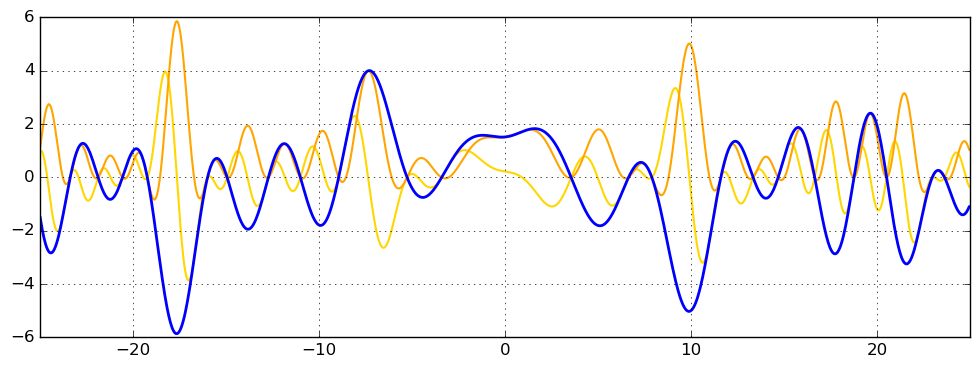This is the L-function for the character modulo 101 with number 11 (index 13) -- LMFDB page for $\chi_{101}(11,\cdot)$:This is the L-function for the character modulo 1009 with number 101 (index 436) -- LMFDB page for $\chi_{1009}(101,\cdot)$: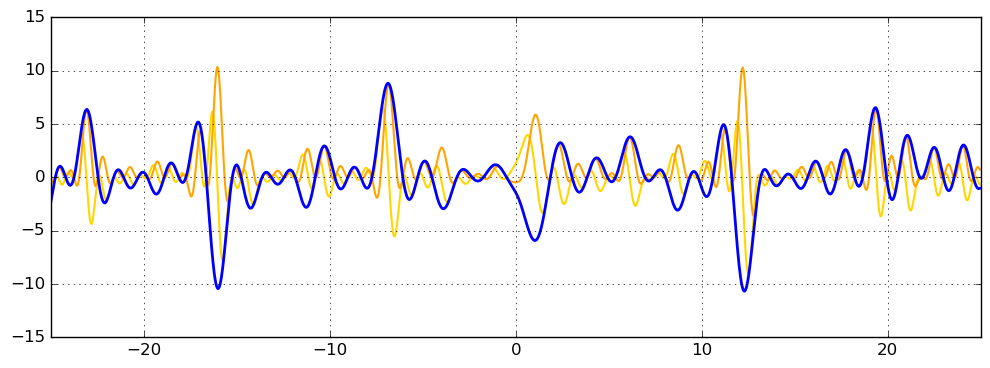This is the L-function for the character modulo 10007 with number 1009 (index 4673) -- it is not yet tabulated in the LMFDB: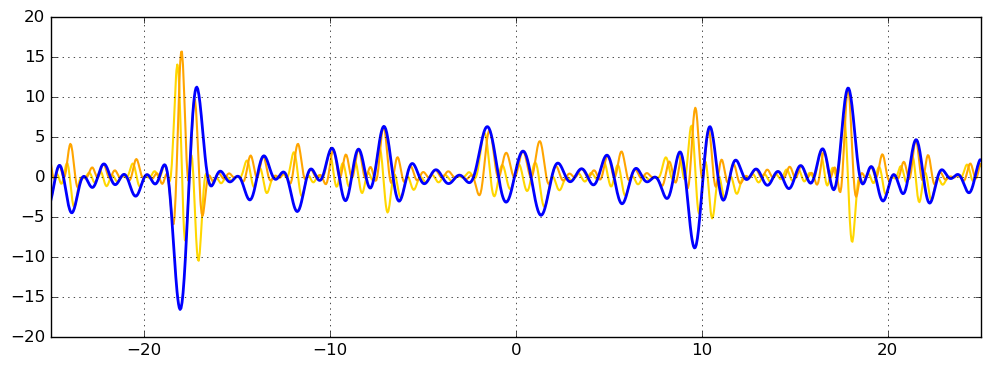One more, modulo 100003 with number 10007 (index 26209).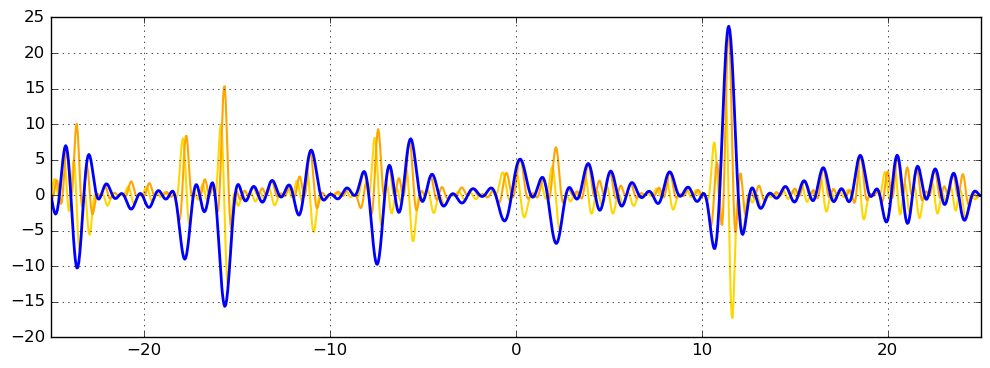Here is a plot of the Z-functions for all 99 primitive Dirichlet L-functions modulo $q = 101$, colored from lowest index (blue) to highest index (red).The same 99 functions, but higher along the critical line at $1000 \le t \le 1050$: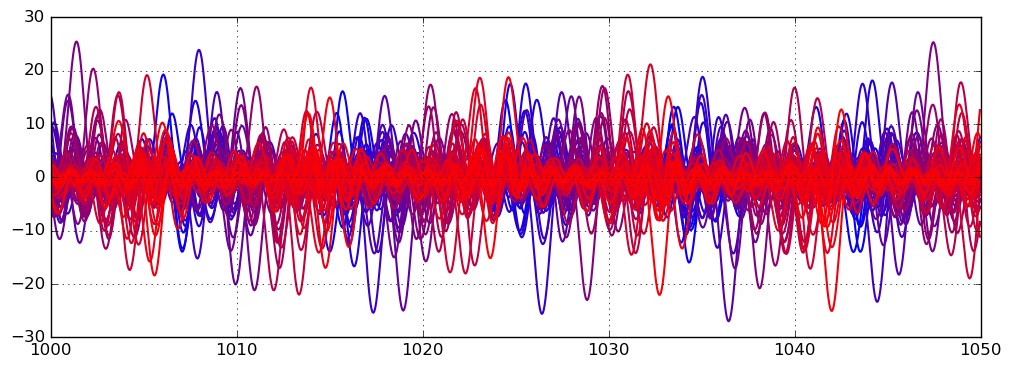This shows the 160 primitive Dirichlet L-functions modulo $q = 1000$: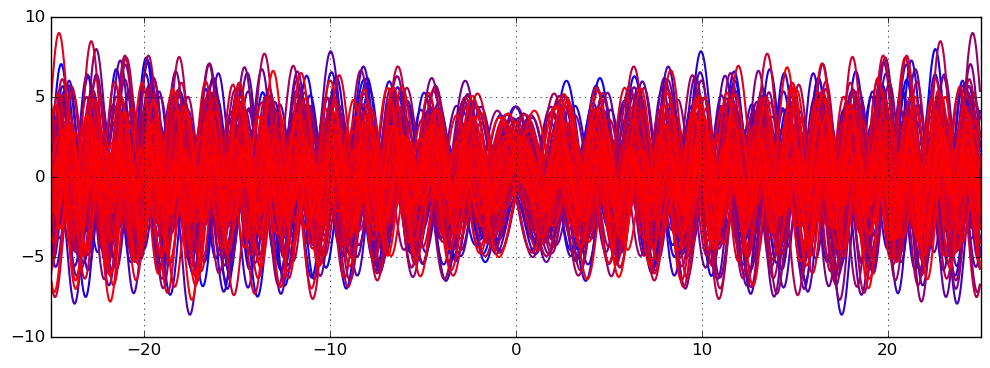We have $\theta(t) = -(t/2) \log(\pi/q) + \operatorname{im}(\log \Gamma(1/4 + it/2 + \delta/2)) + O(1)$, and $\cos(\theta(t))$ roughly captures the average oscillation rate clearly visible in the plots. The behavior around $t = 0$ is interesting: it is actually an open problem whether $L(1/2) \ne 0$ (that is, $Z(0) \ne 0$) for every primitive Dirichlet character.

It is also possible to render Dirichlet L-functions in the complex plane by making simple changes to the complex_plot.c example program included with Arb. This is a picture of the 11.2 L-function on $s = [-10,10] + [-10,30] i$ (rotated 90 degrees for a better viewing experience):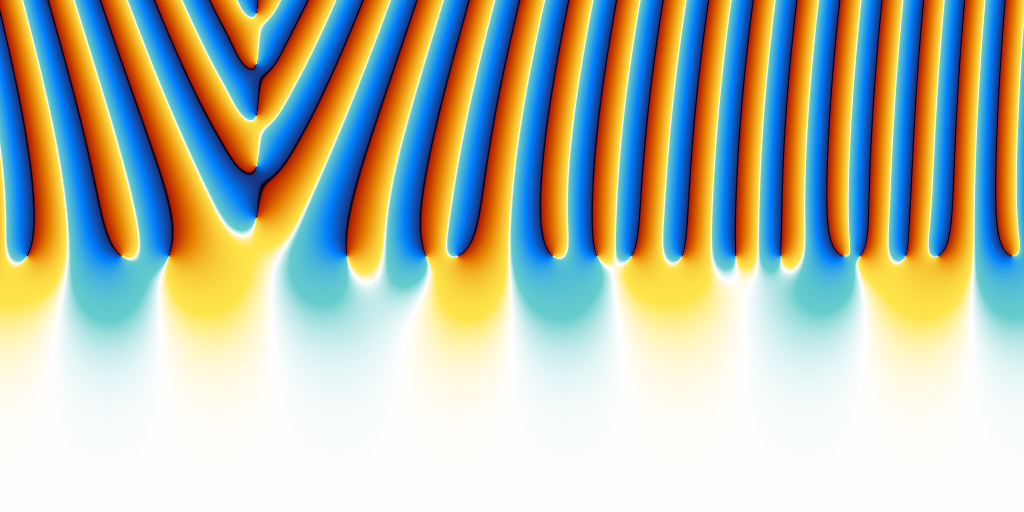## Locating zeros

I have added support for calling Z-functions in the real_roots.c example program included with Arb, so you can easily try out computing the first few zeros of your favorite Dirichlet L-function. Here are all the zeros with $|t| < 10$ of the Z-function corresponding to character number 2 modulo $q = 11$:

$build/examples/real_roots 0 -10 10 -character 11 2 -verbose interval: [-10, 10] maxdepth = 30, maxeval = 100000, maxfound = 100000, low_prec = 30 found isolated root in: [-8.955078125, -8.9453125] found isolated root in: [-5.2734375, -5.2685546875] found isolated root in: [-3.41796875, -3.4130859375] found isolated root in: [3.544921875, 3.5546875] found isolated root in: [6.6259765625, 6.630859375] found isolated root in: [7.880859375, 7.890625] --------------------------------------------------------------- Found roots: 6 Subintervals possibly containing undetected roots: 0 Function evaluations: 6475 cpu/wall(s): 2.676 2.674 virt/peak/res/peak(MB): 26.84 26.86 2.82 2.82  Beware that real_roots.c just uses brute force interval subdivision to prove that no real roots are missed. This gets very slow for modestly large$q$and/or$t$(note that 6475 function evaluations were needed to search$[-10,10]$even though there are only 6 sign changes). There are far more efficient ways (e.g. Turing's method) to provably locate zeros of L-functions and also make sure that there are no zeros off the critical line, which might be implemented in Arb in the future. A relatively simple improvement would be to use Taylor polynomials. Once a zero has been found, refining it to high precision with Newton iteration works very nicely. Here is one zero to 1000 digits: $ build/examples/real_roots 0 3.54 3.55 -character 11 2 -refine 1000
interval: [3.54, 3.55]
maxdepth = 30, maxeval = 100000, maxfound = 100000, low_prec = 30
refined root (0/1):
[3.54704109171945007666447637176567386156297027427569667018019768887902956203532348060236543444891727496733034139321693192555310530877605721402181454776007844101053075911370704878695703773688226743478195049863409292153487721530918396293093590251807278696277730960191843983491195295387137804641541651653604691947113068342761779357720224599209365568315021423832023511365743795411563501473656074161393875006505556918161017146602922835525663710559658303298207284597864073048878314895582790680007858540139547320065032215147599945266935972801502286034406753863137488711128304096999804675297873627974673703596096964906527213943503854853814240528584036172284621208785537337465827483161452388524377901466764361056343144975316692370047616338970126694931010423325588948104132128585464812416817644585087595307816057896430461628124911782958595693022452820462345212075886587009847223863602109249850139699475421920441847031500982858614852157820238432368805828920673340686200909704879310143409822356350849480557479523469 +/- 4.41e-1002]

---------------------------------------------------------------
Found roots: 1
Subintervals possibly containing undetected roots: 0
Function evaluations: 27
cpu/wall(s): 1.469 1.468
virt/peak/res/peak(MB): 27.10 27.10 3.33 3.33


## L-function special values

Dirichlet L-functions have interesting values at special points. Consider the nontrivial Dirichlet character modulo $q = 4$:

#include "dirichlet.h"
#include "acb_dirichlet.h"

int main()
{
dirichlet_group_t G;
dirichlet_char_t chi;
acb_t z, s;
slong k, prec;

acb_init(z); acb_init(s);
dirichlet_group_init(G, 4);
dirichlet_char_init(chi, G);
dirichlet_char_index(chi, G, 1);
prec = 100;

for (k = -10; k <= 10; k++)
{
acb_set_si(s, k);
acb_dirichlet_l(z, s, G, chi, prec);
printf("L(%ld) = ", k);
acb_printn(z, 30, 0); printf("\n");
}

dirichlet_group_clear(G);
dirichlet_char_clear(chi);
acb_clear(z); acb_clear(s);
}


This outputs:

L(-10) = [-25260.50000000000000000000000 +/- 1.59e-24]
L(-9) = 0
L(-8) = [692.5000000000000000000000000 +/- 4.20e-26]
L(-7) = 0
L(-6) = [-30.50000000000000000000000000 +/- 1.77e-27]
L(-5) = 0
L(-4) = [2.500000000000000000000000000 +/- 1.38e-28]
L(-3) = 0
L(-2) = [-0.5000000000000000000000000000 +/- 2.69e-29]
L(-1) = 0
L(0) = [0.500000000000000000000000000000 +/- 6.89e-31]
L(1) = [0.78539816339744830961566084582 +/- 1.76e-30]
L(2) = [0.91596559417721901505460351493 +/- 2.53e-30]
L(3) = [0.96894614625936938048363484585 +/- 4.69e-30]
L(4) = [0.98894455174110533610842263323 +/- 3.53e-30]
L(5) = [0.99615782807708806400631936863 +/- 1.87e-30]
L(6) = [0.99868522221843813544160078786 +/- 1.69e-30]
L(7) = [0.99955450789053990949634654990 +/- 2.27e-30]
L(8) = [0.99984999024682965633806705924 +/- 1.71e-30]
L(9) = [0.99994968418722008982135887329 +/- 4.35e-30]
L(10) = [0.99998316402619687740554072996 +/- 4.13e-30]


The values of this function at the negative integers are the Euler numbers divided by two, $L(-k) = E_k / 2$. The perceptive reader will recognize $L(1) = \pi / 4$, and $L(2)$ is the somewhat famous Catalan's constant. At positive odd integers, $L(2k+1) = (-1)^k E_{2k} \pi^{2k+1} / (4^{k+1} (2k)!)$. For large positive $k$, the Euler product for $L(2k+1)$ converges rapidly, so this formula can be used to compute good numerical approximations of Euler numbers. By computing to high enough precision, the exact values can be deduced. In fact, Arb has a dedicated function for computing Euler numbers which uses this algorithm (computing $E_{100000} \approx 3.7 \cdot 10^{436961}$ exactly takes six seconds).